Question

# A 5.0 kg crate is resting on a horizontal plank. The coefficient of static friction is...

A 5.0 kg crate is resting on a horizontal plank. The coefficient of static friction is 0.50 and the coefficient of kinetic friction is 0.40. One end of the plank is raised so the plank makes an angle of 30° with the horizontal. Find the minimum force that can be applied parallel to the plane to hold the crate at rest.

a. 4.49N

b. 0

c. 2.29N

d. 3.29N

e. 5.59N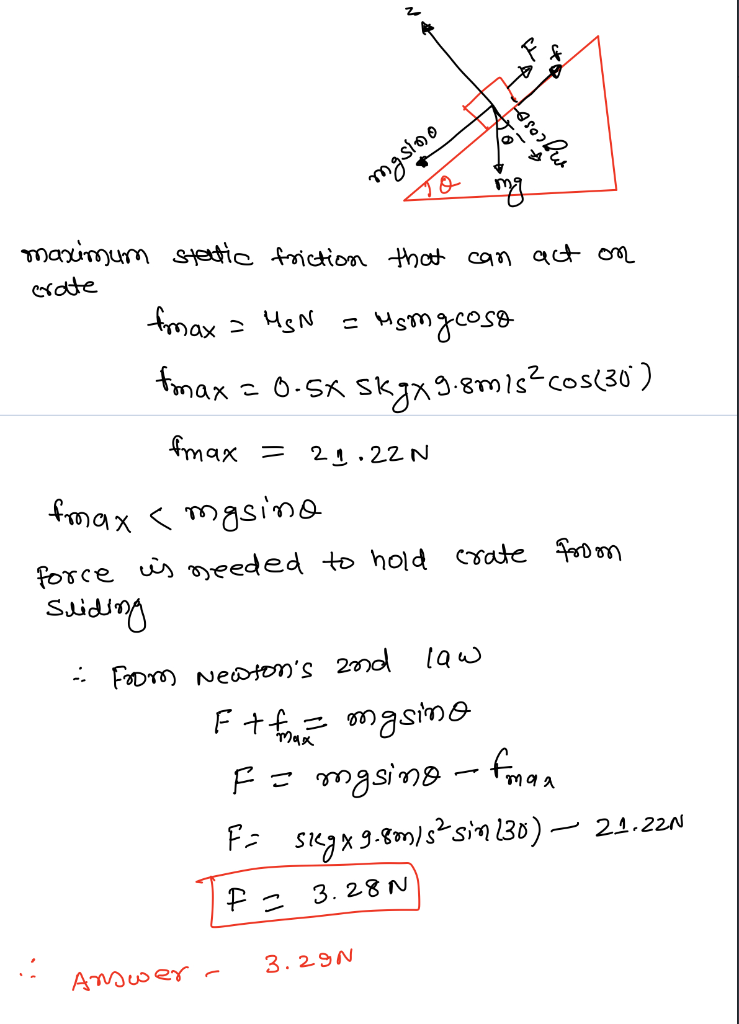#### Earn Coins

Coins can be redeemed for fabulous gifts.

Similar Homework Help Questions
• ### help pls A horizontal force pushes on a 50 kg crate resting on the floor. Once...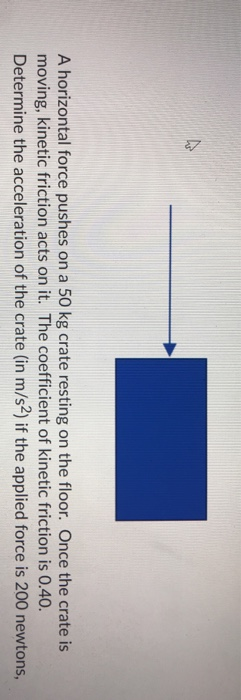help pls A horizontal force pushes on a 50 kg crate resting on the floor. Once the crate is moving, kinetic friction acts on it. The coefficient of kinetic friction is 0.40. Determine the acceleration of the crate (in m/s) if the applied force is 200 newtons,

• ### Figure 5 shows a crate has a mass of 50 kg and the coefficient of static...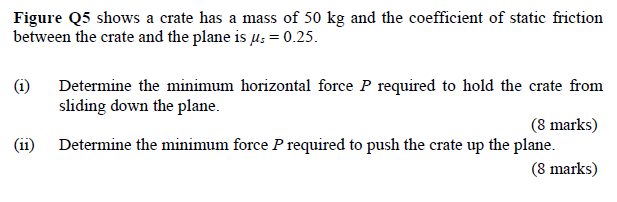Figure 5 shows a crate has a mass of 50 kg and the coefficient of static friction between the crate and the plane is us = 0.25. (1) Determine the minimum horizontal force P required to hold the crate from sliding down the plane. (8 marks) Determine the minimum force P required to push the crate up the plane. (8 marks) (11) P 30° Figure Q5

• ### Problem 04 ed A 244-kg crate is being above the horizontal. The coefficient of kinetic friction...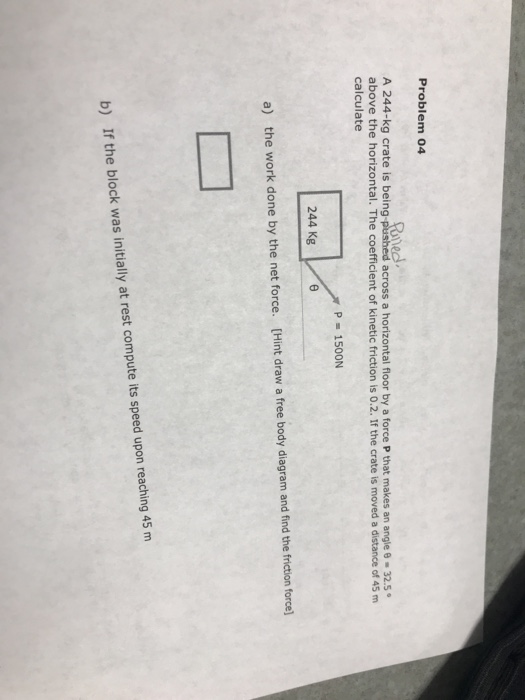Problem 04 ed A 244-kg crate is being above the horizontal. The coefficient of kinetic friction is 0.2. If the crate is moved a distance of 45 m calculate across a horizontal floor by a force P that makes an angle 8-32.5 P 150ON 244 Kg [Hint draw a free body diagram and find the friction force] a) the work done by the net force. b) If the block was initially at rest compute its speed upon reaching 45 m

• ### If you push with a horizontal 650-N force to a 90-kg crate initially at resting on...

If you push with a horizontal 650-N force to a 90-kg crate initially at resting on the floor, what must acceleration be? The static and kinetic energy coefficient of friction are us- 0.4, uk= 0.25 respectively.

• ### A mass of 9 kg rests on a floor with a coefficient of static friction of...

A mass of 9 kg rests on a floor with a coefficient of static friction of 1.25 and coefficient of kinetic friction of kinetic friction of 0.1. One force is applied vertically downward of 10 Newtons and another force is applied at an angle of 15 degrees below the horizontal. Calculate what the magnitude this force needs to be in order to begin to move the mass. If the same amount of force is applied at the same angle above...

• ### 1. A 500 kg wooden crate is on a wooden floor.The coefficient of static friction is...

1. A 500 kg wooden crate is on a wooden floor.The coefficient of static friction is 0.3. What is the minimum magnitude of a horizontal force needed to start it moving?

• ### A 500 kg wooden crate is on a wooden floor.The coefficient of static friction is 0.3....

A 500 kg wooden crate is on a wooden floor.The coefficient of static friction is 0.3. What is the minimum magnitude of a horizontal force needed to start it moving? THIS QUESTION: What for is needed to keep the crate in question # 1 moving?

• ### The coefficient of static friction between the 3.00 kg crate and the 33 degree incline of...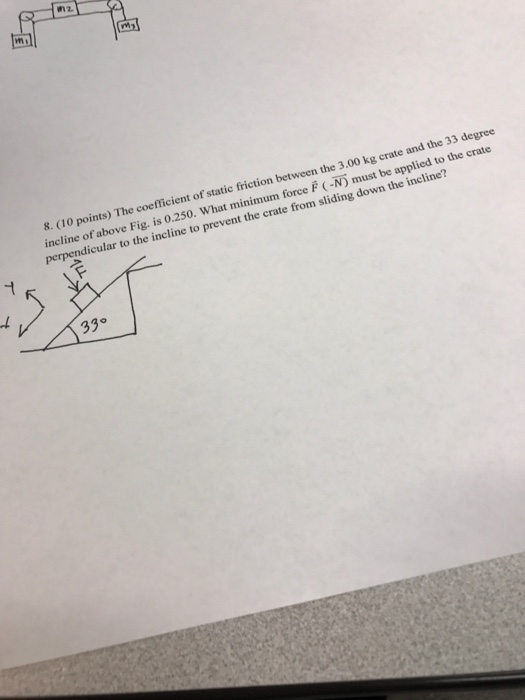The coefficient of static friction between the 3.00 kg crate and the 33 degree incline of above Fig. is 0.250. What minimum force F(-N) must be applied to the crate perpendicular to the incline to prevent the crate form sliding down the incline?

• ### The coefficient of static friction between the m = 3.85-kg crate and the 35.0° incline of...

The coefficient of static friction between the m = 3.85-kg crate and the 35.0° incline of the figure below is 0.325. What minimum force F must be applied to the crate perpendicular to the incline to prevent the crate from sliding down the incline? N

• ### A 39 kg crate sits on a horizontal floor. The coefficients of static and kinetic friction...

A 39 kg crate sits on a horizontal floor. The coefficients of static and kinetic friction between the crate and floor are 0.6 and 0.4, respectively. Use a coordinate system with +y pointing upwards. a) How much force do you need to apply to get the crate sliding along the floor? [  ,  ,  ] N b) If the crate is moving with a speed of [0.8, 0, 0] m/s, what force is it experiencing due to friction as it slides? [  ,  ,  ] N...